2018-12-07 17:35:38 RedPintings 阅读数 1602
• ###### Python入门视频精讲

Python入门视频培训课程以通俗易懂的方式讲解Python核心技术，Python基础，Python入门。适合初学者的教程，让你少走弯路！ 课程内容包括：1.Python简介和安装 、2.第一个Python程序、PyCharm的使用 、3.Python基础、4.函数、5.高级特性、6.面向对象、7.模块、8.异常处理和IO操作、9.访问数据库MySQL。教学全程采用笔记+代码案例的形式讲解，通俗易懂！！！

8447 人正在学习 去看看 汤小洋

Python在大数据行业非常火爆近两年，as a pythonic，所以也得涉足下大数据分析，下面就聊聊它们。

Python数据分析与挖掘技术概述

1. numpy 高效处理数据，提供数组支持，很多模块都依赖它，比如pandas，scipy，matplotlib都依赖他，所以这个模块都是基础。所以必须先安装numpy。
2. pandas 主要用于进行数据的采集与分析
3. scipy 主要进行数值计算。同时支持矩阵运算，并提供了很多高等数据处理功能，比如积分，微分方程求样等。
4. matplotlib 作图模块，结合其他数据分析模块，解决可视化问题
5. statsmodels 这个模块主要用于统计分析
6. Gensim 这个模块主要用于文本挖掘
7. sklearn，keras 前者机器学习，后者深度学习。

numpy模块安装与使用

numpy简单使用

``````import numpy

x=numpy.array([11,22,33,4,5,6,7,])  #创建一维数组
x2=numpy.array([['asfas','asdfsdf','dfdf',11],['1iojasd','123',989012],["jhyfsdaeku","jhgsda"]])    #创建二维数组,注意是([])

x.sort()   #排序，没有返回值的，修改原处的值，这里等于修改了X
x.max()    # 最大值，对二维数组都管用
x.min()    # 最小值，对二维数组都管用
x1=x[1:3]   # 取区间，和python的列表没有区别``````

``````#numpy.random.random_integers(最小值,最大值,个数)  获取的是正数
data = numpy.random.random_integers(1,20000,30)   #生成整形随机数
#正态随机数  numpy.random.normal(均值,偏离值,个数)  偏离值决定了每个数之间的差 ,当偏离值大于开始值的时候，那么会产生负数的。
data1 = numpy.random.normal(3.2,29.2,10)    # 生成浮点型且是正负数的随机数``````

pandas

``````print(b)
0     1     2    3
0     1     2     3  4.0
1  sdaf  dsaf  18hd  NaN
2  1463  None  None  NaN``````

``````import pandas
a=pandas.Series([1,2,3,34,])   # 等于一维数组
b=pandas.DataFrame([[1,2,3,4,],["sdaf","dsaf","18hd"],])   # 二维数组
print(b.tail())   # 默认取尾部前后5行
print(b.tail(1))     # 直接传入参数，如我写的那样``````

``````# print(b.describe())   # 显示统计数据信息
3      # 3表示这个二维数组总共多少个元素
count  1.0      # 总数
mean   4.0      # 平均数
std    NaN      # 标准数
min    4.0      # 最小数
25%    4.0      # 分位数
50%    4.0      # 分位数
75%    4.0      # 分位数
max    4.0      # 最大值``````

``````print(b.T)   # 转置
0     1     2
0  1  sdaf  1463
1  2  dsaf  None
2  3  18hd  None
3  4   NaN   NaN``````

pandas支持多种输入格式，我这里就简单罗列日常生活最常用的几种，对于更多的输入方式可以查看源码后者官网。

CSV文件

csv文件导入后显示输出的话，是按照csv文件默认的行输出的，有多少列就输出多少列，比如我有五列数据，那么它就在prinit输出结果的时候，就显示五列

``````csv_data = pandas.read_csv('F:\Learnning\CSDN-python大数据\hexun.csv')
print(csv_data)``````

excel表格

``````excel_data = pandas.read_excel('F:\Learnning\CSDN-python大数据\cxla.xls')
print(excel_data)``````

``````conn=pymysql.connect(host="127.0.0.1",user="root",passwd="root",db="test")
sql="select * from fortest"

``````html_data = pandas.read_html('F:\Learnning\CSDN-python大数据\shitman.html')   # 读取本地html文件。
print(html_data)
print('html_from_online')``````

``````text_data = pandas.read_table('F:\Learnning\CSDN-python大数据\dforsay.txt')
print(text_data)``````

scipy

matplotlib 数据可视化分析

``````from matplotlib import  pylab
import numpy
# 下面2行定义X轴，Y轴
x=[1,2,3,4,8]
y=[1,2,3,4,8]
# plot的方法是这样使用（x轴数据,y轴数据,展现形式）
pylab.plot(x,y)   # 先把x,y轴的信息塞入pylab里面，再调用show方法来画图
pylab.show()    # 这一步开始画图,默认是至线图``````1. 直线图（默认）
2. `-` 直线
3. -- 虚线
4. -. -.形式
5. : 细小虚线

1. c-青色
2. r-红色
3. m-品红
4. g-绿色
5. b-蓝色
6. y-黄色
7. k-黑色
8. w-白色

1. s 方形
2. `*` 星形
3. p 五角形

``````pylab.plot(x,y,'or')   # 添加O表示画散点图，r表示red
pylab.show()````````````pylab.plot(x,y,'r:')
pylab.show()``````

``````pylab.plot(x,y,'pr--')   #p是图形为五角星，r为红色，--表示虚线
pylab.title('for learnning')   # 图形标题
pylab.xlabel('args')   # x轴标签
pylab.ylabel('salary')   # y轴标签
pylab.xlim(2)    # 从y轴的2开始做线
pylab.show()````````````data1 = numpy.random.normal(5.0,4.0,10)   # 正态随机数
pylab.hist(data1)
pylab.show()``````Y轴为出现的次数，X轴为这个数的值（或者是范围）

1. bar ：is a traditional bar-type histogram. If multiple data
are given the bars are aranged side by side.
2. barstacked ：is a bar-type histogram where multiple
data are stacked on top of each other.
3. step ：generates a lineplot that is by default
unfilled.
4. stepfilled ：generates a lineplot that is by default
filled.

``````sty=numpy.arange(1,30,2)
pylab.hist(data1,histtype='stepfilled')
pylab.show() ````````````#subplot(行,列,当前区域)
pylab.subplot(2,2,1)   # 申明一个大图里面划分成4块（即2*2），子图使用第一个区域（坐标为x=1,y=1）
pylab.subplot(2,2,2)  # 申明一个大图里面划分成4块（即2*2），子图使用第二个区域（坐标为x=2,y=2）
x1=[1,4,6,9]
x2=[3,21,33,43]
pylab.plot(x1,x2)   # 这个plot表示把x,y轴数据塞入前一个子图中。我们可以在每一个子图后使用plot来塞入x,y轴的数据
pylab.subplot(2,1,2)  # 申明一个大图里面划分成2块（即），子图使用第二个区域（坐标为x=1,y=2）
pylab.show()``````### 实践小例子

``````
dt = csv_data.T   # 装置下，把阅读数和评论数转为行
pylab.show()``````python大数据入门 相关内容

2018-12-20 16:02:29 zwx19921215 阅读数 670
• ###### Python入门视频精讲

Python入门视频培训课程以通俗易懂的方式讲解Python核心技术，Python基础，Python入门。适合初学者的教程，让你少走弯路！ 课程内容包括：1.Python简介和安装 、2.第一个Python程序、PyCharm的使用 、3.Python基础、4.函数、5.高级特性、6.面向对象、7.模块、8.异常处理和IO操作、9.访问数据库MySQL。教学全程采用笔记+代码案例的形式讲解，通俗易懂！！！

8447 人正在学习 去看看 汤小洋

python数据分析入门，作为入门文章系列主要包含以下几个内容：

1.数据的来源（本案例采用的数据来自于上一篇文章中爬取的智联招聘信息）：读取数据库数据、数据写入csv文件、读取csv文件等

2.数据的处理：对载入到内存的数据进行一系列的操作（处理总共包含清洗、过滤、分组、统计、排序、计算平均数等一系列操作，本文只简单涉及了其中某几个）

3.对处理后的数据进行可视化分析等

``````# ！/usr/bin/env python
# -*-coding:utf-8-*-
"""
@Author  : xiaofeng
@Time    : 2018/12/19 15:23
@Desc : Less interests,More interest.（数据分析入门）
@Project : python_appliction
@FileName: analysis1.py
@Software: PyCharm
@Blog    ：https://blog.csdn.net/zwx19921215
"""
import pymysql as db
import pandas as pd
import numpy as np
import seaborn as sns
import matplotlib as mpl
import matplotlib.pyplot as plt

# mysql config
mysql_config = {
'host': '110.0.2.130',
'user': 'test',
'database': 'xiaofeng',
'charset': 'utf8'
}

"""
read data from mysql and write to local file
（从数据库中读取数据写入本地文件）
@:param page_no
@:param page_size
@:param path 文件写入路径
"""

database = db.connect(**mysql_config)
if page_no > 1:
page_no = (page_no - 1) * page_size
else:
page_no = 0
sql = 'select * from zhilian limit ' + str(page_no) + ',' + str(page_size) + ''
print(df)
database.close()
# write to csv
local = path
df.to_csv(local, encoding='gbk')

"""
（读取文件从远程地址或者本地）
"""

# data_url = 'https://xxxx/zhilian.csv'
# data_url = 'G:/zhilian.csv'
return df

"""

"""

def simple_op(path):
# top 10 ：获取前10行
print('--------------top 10-----------')
print(top)
# tail 10：获取后10行
print('------------tail 10------------')
tail = df.tail(10)
print(tail)
# filter：根据指定条件过滤
print('-------------filter----------')
special_jobid = df[df.JobID == 595950]
print(special_jobid)
special = df[(df.id >= 110) & (df.PublishDate == '2018-12-18')]
print(special)
# check ：检查各列缺失情况
print('-------------check----------------')
check = df.info()
print(check)
print('--------------data describe-----------')
# count，平均数，标准差，中位数，最小值，最大值，25 % 分位数，75 % 分位数
describe = df.describe()
print(describe)
# 添加新列
df2 = df.copy()
df2['AnnualSalaryAvg'] = (df['AnnualSalaryMax'] + df['AnnualSalaryMin']) / 2
# 重新排列指定列
columns = ['JobID', 'JobTitle', 'CompanyName', 'AnnualSalaryMin', 'AnnualSalaryMax', 'AnnualSalaryAvg']
df = pd.DataFrame(df2, columns=columns)
print(df)

"""

"""

def visualized_analysis(path):
df_post_count = df.groupby('JobLactionStr')['AnnualSalaryMax'].count().to_frame().reset_index()
# subplots(1, 1) 表示1x1个子图，figsize=(25, 8) 子图的宽度和高度
# f, [ax1,ax2] = plt.subplots(1, 2, figsize=(25, 8)) 表示1x2个子图
f, ax2 = plt.subplots(1, 1, figsize=(25, 8))

sns.barplot(x='JobLactionStr', y='AnnualSalaryMax', palette='Greens_d', data=df_post_count, ax=ax2)
ax2.set_title('各城市职位数量对比', fontsize=15)
ax2.set_xlabel('城市')
ax2.set_ylabel('数量')
# 用来正常显示中文标签
plt.rcParams['font.sans-serif'] = ['SimHei']
# 用来正常显示负号
plt.rcParams['axes.unicode_minus'] = False
plt.show()

if __name__ == '__main__':
path = 'G:/zhilian.csv'
simple_op(path)
visualized_analysis(path)
``````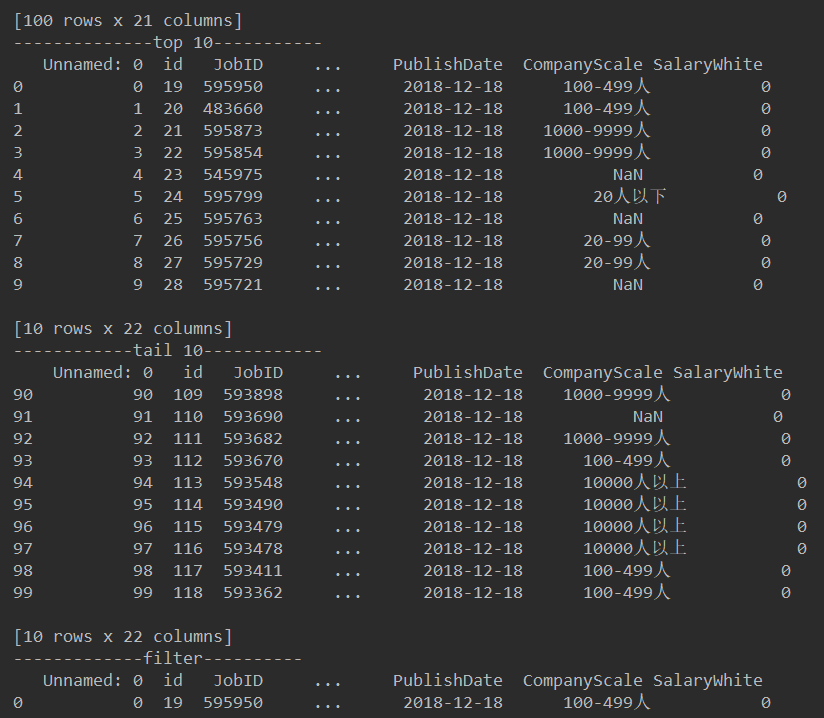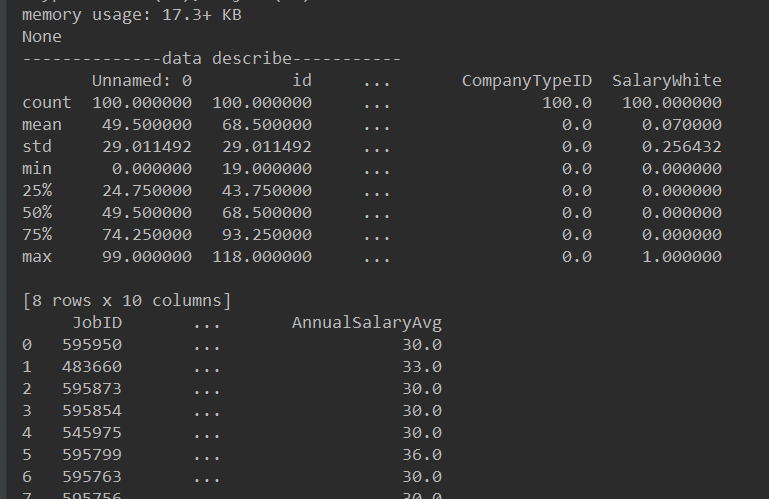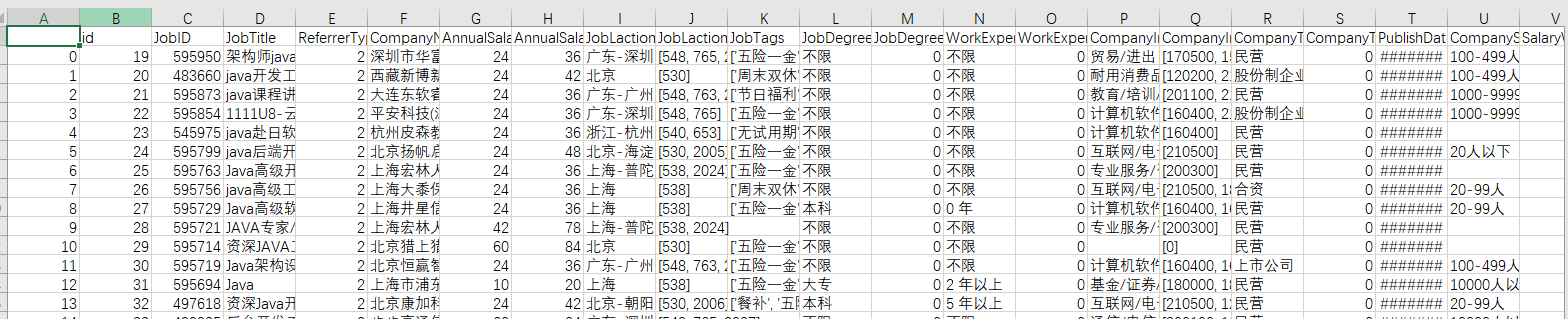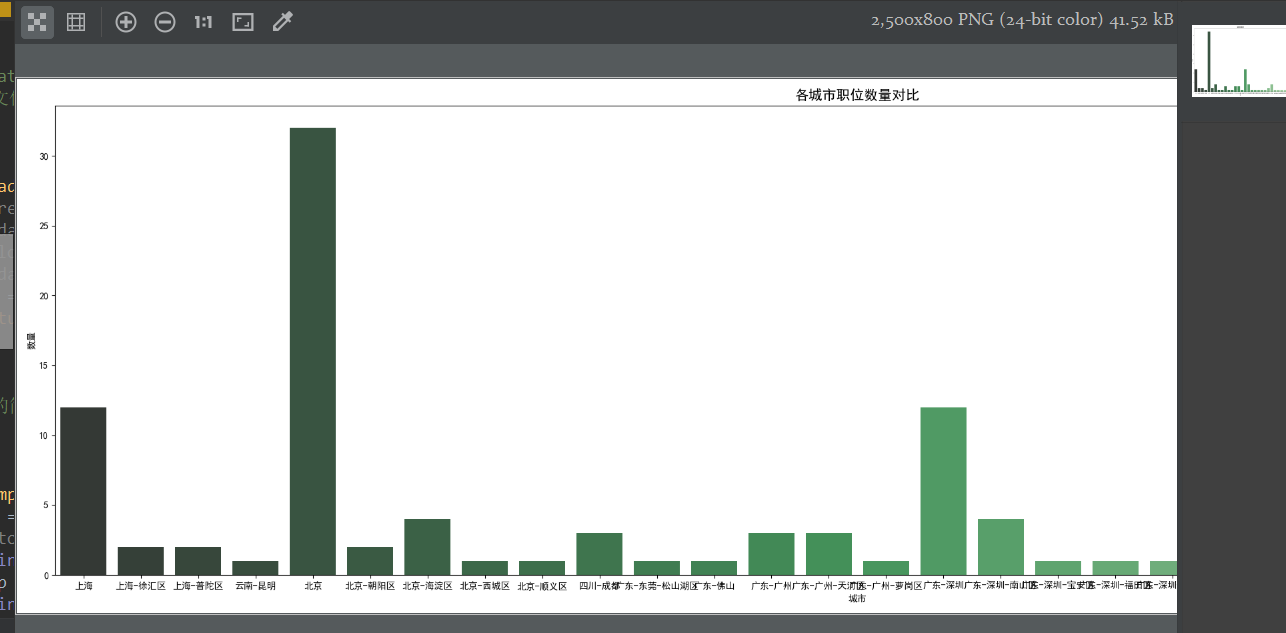python大数据入门 相关内容

2018-05-15 22:04:17 qq_41597912 阅读数 802
• ###### Python入门视频精讲

Python入门视频培训课程以通俗易懂的方式讲解Python核心技术，Python基础，Python入门。适合初学者的教程，让你少走弯路！ 课程内容包括：1.Python简介和安装 、2.第一个Python程序、PyCharm的使用 、3.Python基础、4.函数、5.高级特性、6.面向对象、7.模块、8.异常处理和IO操作、9.访问数据库MySQL。教学全程采用笔记+代码案例的形式讲解，通俗易懂！！！

8447 人正在学习 去看看 汤小洋Pandas提供了很多针对数据的操作，本文列出一些非常常用的。

# 实战练习

import pandas as pd

df = pd.DataFrame({'col1':[1,2,3,4],'col2':[444,555,666,444],'col3':['abc','def','ghi','xyz']})df['col2'].unique()df['col2'].nunique()df['col2'].value_counts()

newdf = df[(df['col1']>2) & (df['col2']==444)]

newdfdef times2(x):

return x*2

df['col1'].apply(times2)df['col2'].apply(lambda x:x*2)df['col3'].apply(len)df['col1'].sum()del df['col1']

dfdf = pd.DataFrame({'col1':[1,2,3,4],'col2':[444,555,666,444],'col3':['abc','def','ghi','xyz']})

df.drop('col1',axis=1,inplace=True)

dfdf.columnsdf.indexdf

df.sort_values(by='col2') #inplace=False by defaultdf.isnull()df.dropna()import numpy as np

df = pd.DataFrame({'col1':[1,2,3,np.nan],

'col2':[np.nan,555,666,444],

'col3':['abc','def','ghi','xyz']})df.fillna('FILL')data = {'A':['foo','foo','foo','bar','bar','bar'],

'B':['one','one','two','two','one','one'],

'C':['x','y','x','y','x','y'],

'D':[1,3,2,5,4,1]}

df = pd.DataFrame(data)

df# 进度介绍

1. 预热

2. 环境搭建

3. Jupyter教程

4. Python速成

5. Python数据分析，NumPy库的使用

6. Python数据分析，Pandas库的使用

7. Python数据分析，Pandas库练习

8. Python数据可视化，Matplotlib

9. Python数据可视化，Seaborn

10. Python数据可视化，Pandas内建数据可视化

12. Python数据可视化，Geographical Plotting

13. 数据 Capstone 项目

14. 机器学习介绍

15. 线性回归

16. 交叉验证与偏方差

17. 逻辑回归算法

18. k-近邻算法

19. 决策树与随机森林

20. 支持向量机

21. k-means聚类

22. 主成分分析

23. 推荐系统

24. 自然语言处理(NLP)

25. Python大数据与Spark

26. 神经网络（NN）与深度学习（DL）

27.

python大数据入门 相关内容

2019-08-10 12:36:34 weixin_43216249 阅读数 104
• ###### Python入门视频精讲

Python入门视频培训课程以通俗易懂的方式讲解Python核心技术，Python基础，Python入门。适合初学者的教程，让你少走弯路！ 课程内容包括：1.Python简介和安装 、2.第一个Python程序、PyCharm的使用 、3.Python基础、4.函数、5.高级特性、6.面向对象、7.模块、8.异常处理和IO操作、9.访问数据库MySQL。教学全程采用笔记+代码案例的形式讲解，通俗易懂！！！

8447 人正在学习 去看看 汤小洋

## 课节1：Python数据分析处理

### Python数据分析入门

#### 波士顿房价的预测（数据分析和建模的初步知识）（附带相应知识的链接）

##### 一、基础库介绍
###### 1.Seaborn:

Seaborn是基于matplotlib的Python可视化库。 它提供了一个高级界面来绘制有吸引力的统计图形。Seaborn其实是在matplotlib的基础上进行了更高级的API封装，从而使得作图更加容易，不需要经过大量的调整就能使你的图变得精致。但应强调的是，应该把Seaborn视为matplotlib的补充，而不是替代物。

###### 2.XGBoost:

XGBoost可以成为机器学习的大杀器，广泛用于数据科学竞赛和工业界，是因为它有许多优点：

（1.使用许多策略去防止过拟合，如：正则化项、Shrinkage and Column Subsampling等。

（2. 目标函数优化利用了损失函数关于待求函数的二阶导数

（3.支持并行化，这是XGBoost的闪光点，虽然树与树之间是串行关系，但是同层级节点可并行。具体的对于某个节点，节点内选择最佳分裂点，候选分裂点计算增益用多线程并行。训练速度快。

（4.添加了对稀疏数据的处理。

（5.交叉验证，early stop，当预测结果已经很好的时候可以提前停止建树，加快训练速度。

（6.支持设置样本权重，该权重体现在一阶导数g和二阶导数h，通过调整权重可以去更加关注一些样本

###### 3.Sklearn: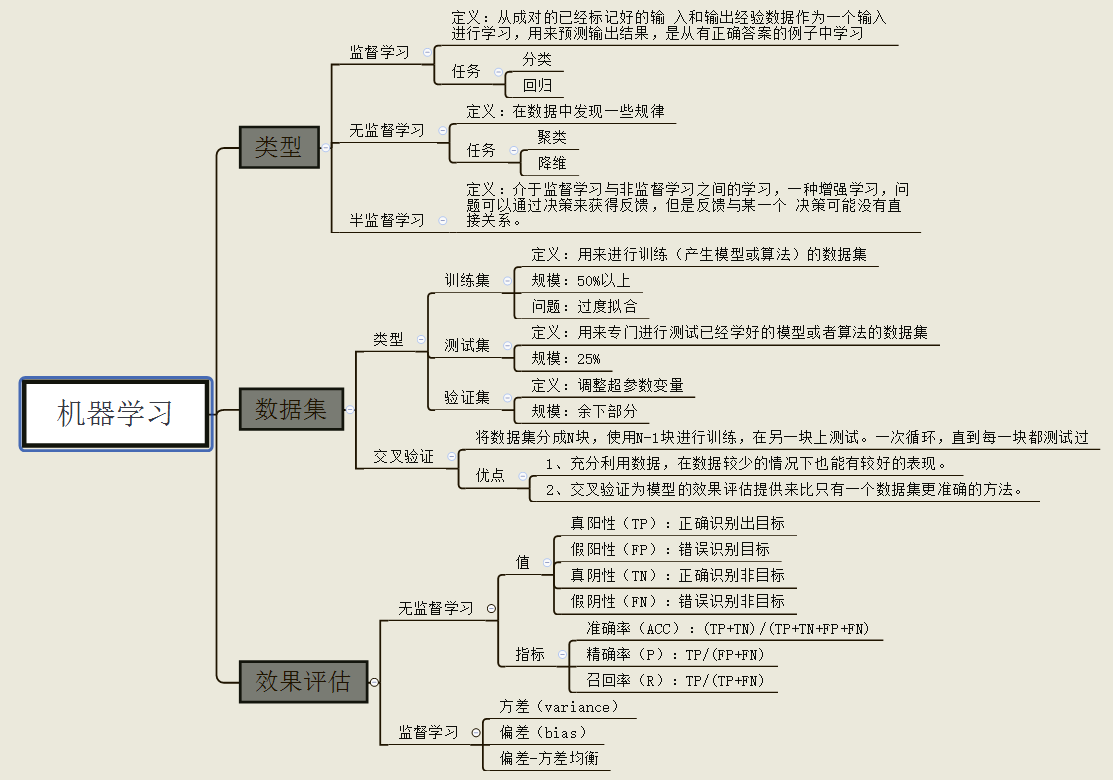###### 5.Matplotlib

Matplotlib 是基于 NumPy 数组构建的多平台数据可视化库。它是John Hunter 在2002年构想的，原本的设计是给 IPython 打补丁，让命令行中也可以有交互式的 MATLAB 风格的画图工具。

http://www.sohu.com/a/318173714_464033

##### 二、代码详情
###### 安装或导入必要的package
``````!pip install seaborn
!pip install xgboost
!pip install sklearn
``````
``````import pandas as pd
import numpy as np
import seaborn as sns
import matplotlib as mpl

import matplotlib.pyplot as plt
from IPython.display import display
plt.style.use("fivethirtyeight")
sns.set_style({'font.sans-serif':['simhei','Arial']})
%matplotlib inline
``````
``````#导入数据
``````
``````display(housing.head(n=5))#显示数据前5个
``````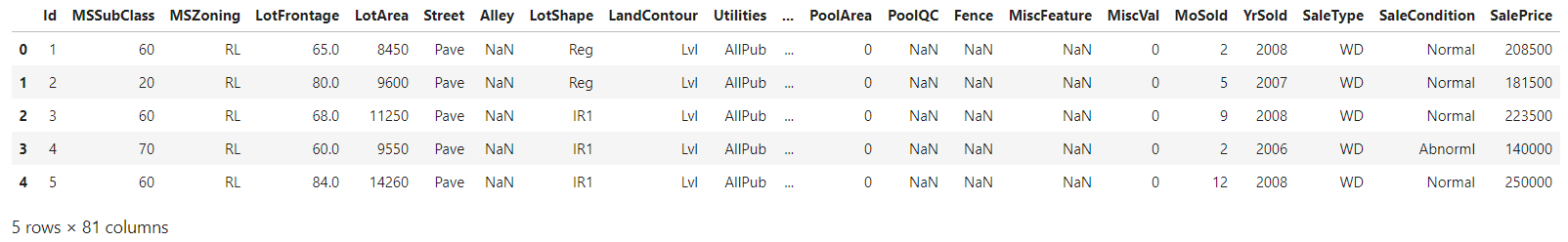``````housing.info()#信息统计
``````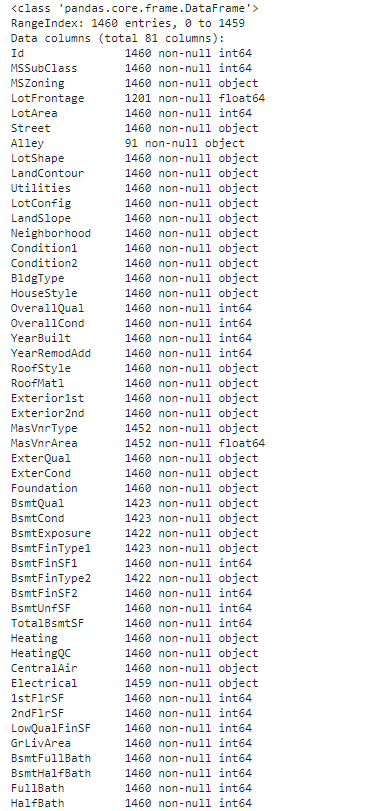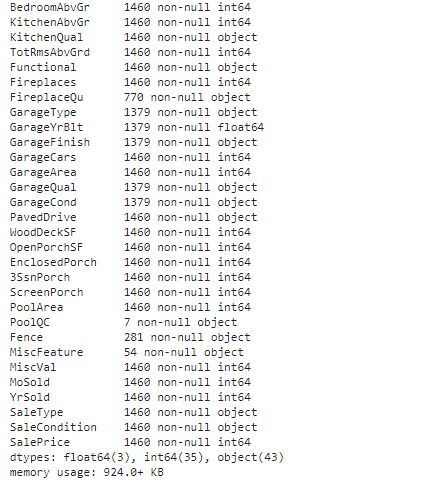``````housing.describe()#信息描述
``````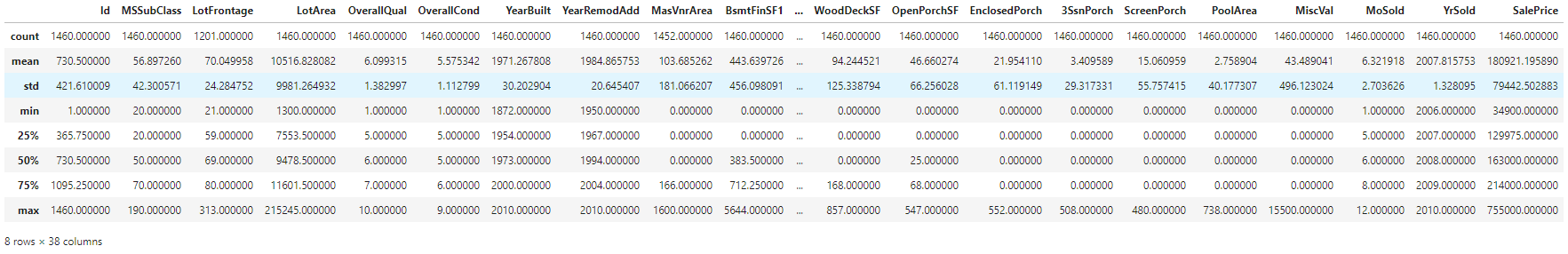###### 可视化处理
``````# 对区域分组对比每平米房价
housing_count = housing.groupby('LotArea')['SalePrice'].count().sort_values(ascending=False).to_frame().reset_index()

f, [ax2,ax3] = plt.subplots(2,1,figsize=(15,15))#几个子图，

sns.barplot(x='LotArea', y='SalePrice', palette="Greens_d", data=housing_count, ax=ax2)#条形图
ax2.set_title('数量',fontsize=15)
ax2.set_xlabel('区域')
ax2.set_ylabel('数量')

sns.boxplot(x='LotArea', y='SalePrice', data=housing, ax=ax3)
ax3.set_title('总价',fontsize=15)
ax3.set_xlabel('区域')
ax3.set_ylabel('房屋总价')

plt.show()
``````

groupby:进行分组，并且进行组内运算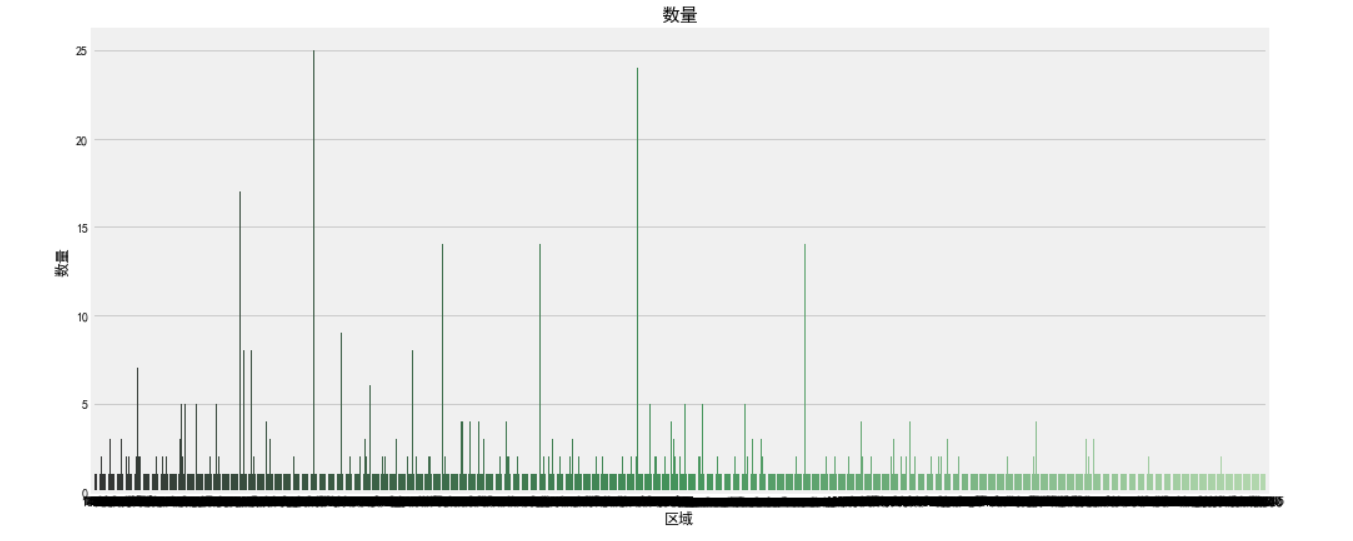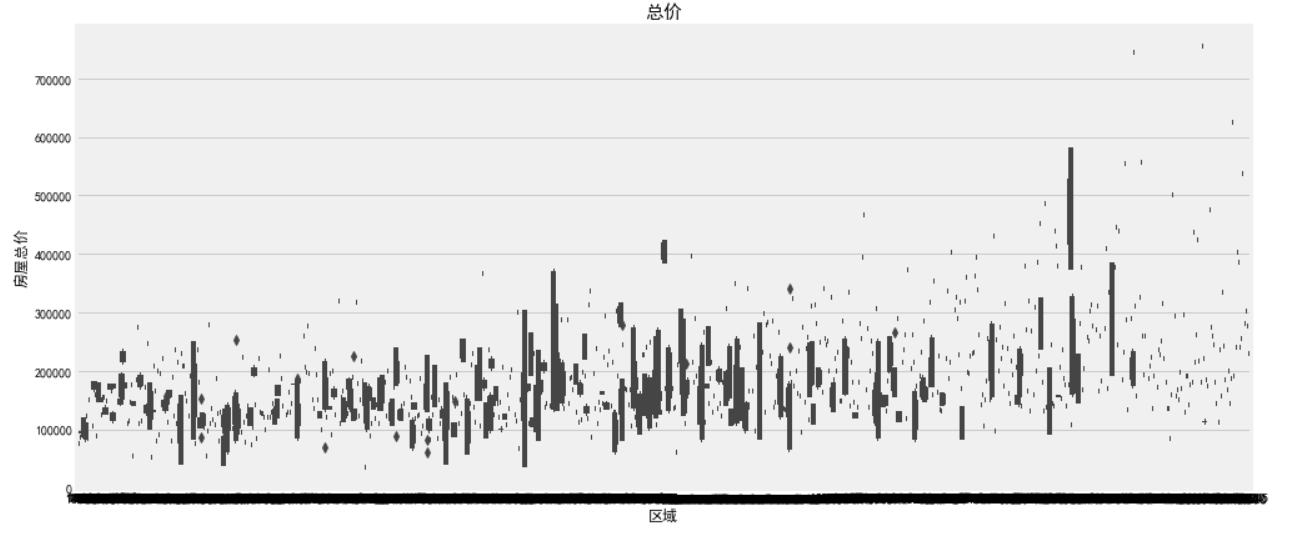``````f, [ax1,ax2] = plt.subplots(1, 2, figsize=(15, 5))#figsize为设定的尺寸
# 建房时间的分布情况
sns.distplot(housing['YearBuilt'], bins=20, ax=ax1, color='r')#displot()集合了matplotlib的hist()与核函数估计kdeplot的功能，增加了rugplot分布观测条显示与利用scipy库fit拟合参数分布的新颖用途。
# 建房时间和出售价格的关系
sns.regplot(x='YearBuilt', y='SalePrice', data=housing, ax=ax2)
plt.show()
``````

subplots详解：https://blog.csdn.net/htuhxf/article/details/82986440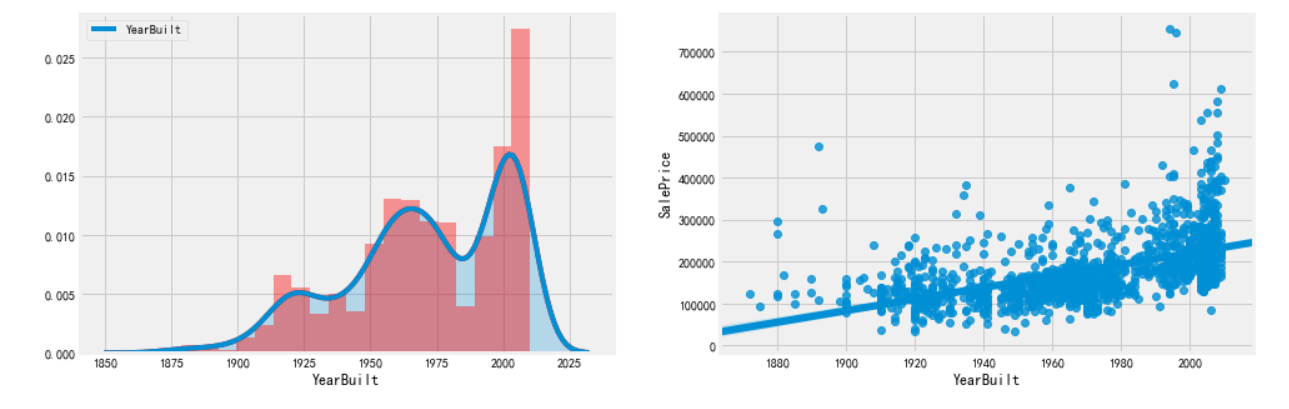``````#缺失值查找
misn = len(housing.loc[(housing['Fence'].isnull()), 'Fence'])
print('Fence缺失值数量为：'+ str(misn))
``````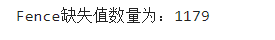loc：通过行标签索引数据

iloc：通过行号索引行数据

ix：通过行标签或行号索引数据（基于loc和iloc的混合）

###### 特征工程

``````import pandas as pd
import numpy as np
from scipy.stats import skew
import xgboost as xgb
from sklearn.model_selection  import KFold
from sklearn.ensemble import ExtraTreesRegressor
from sklearn.ensemble import RandomForestRegressor
from sklearn.metrics import mean_squared_error
from sklearn.linear_model import Ridge, RidgeCV, ElasticNet, LassoCV, Lasso
from math import sqrt
``````
###### 参数设置
``````TARGET = 'SalePrice'
NFOLDS = 5#K-Fold 交叉验证 (Cross-Validation)中的组数
SEED = 0
NROWS = None

ntrain = train.shape#1460
ntest = test.shape#1459
``````
``````y_train = np.log(train[TARGET]+1)
``````
``````train.drop([TARGET],axis = 1, inplace = True)#删除指定范围
``````
``````all_data = pd.concat((train.loc[:,'MSSubClass':'SaleCondition'],
test.loc[:,'MSSubClass':'SaleCondition']))#数据合并与重塑
``````
``````#log transform skewed numeric features
numer_feats = all_data.dtypes[all_data.dtypes != "object"].index
``````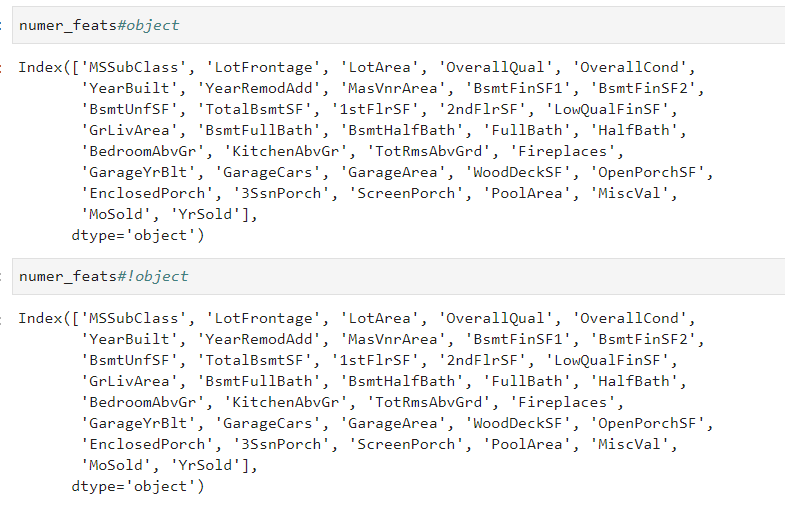``````skewed_feats1 = train[numer_feats].apply(lambda x: skew(x.dropna()))#运用偏度以及过滤缺失值
skewed_feats2 = skewed_feats1[skewed_feats1 > 0.75]#利用偏度缩小范围,设为0.75原因是因为skew 太高的情况下，特征值会有较为严重的 shake up，特征的变化对于 模型的影响很大。这里选的是>0.75，选的大的，可以发现偏得很严重。
skewed_feats3 = skewed_feats2.index#保留skewed_feats2的索引
``````
``````all_data[skewed_feats3] = np.log1p(all_data[skewed_feats3])#平滑化数据，使其符合正态分布
``````
``````all_data = pd.get_dummies(all_data)#哑变量编码,类似于独热编码
``````

dummies详解：https://www.jianshu.com/p/5f8782bf15b1

``````#filling NA's with the mean of the column:
all_data =all_data.fillna(all_data.mean())#将空缺的位置填充平均值
``````
``````#creating matrices for sklearn:
x_train = np.array(all_data[:train.shape])
x_test = np.array(all_data[train.shape:])
``````
``````kf = KFold(NFOLDS,shuffle=True,random_state=SEED)
``````

https://www.jianshu.com/p/284581d9b189

https://blog.csdn.net/GitzLiu/article/details/82670315

https://blog.csdn.net/accumulate_zhang/article/details/78490394

``````class SklearnWrapper(object):
def __init__(self,clf,seed=0,params=None):
params['random_state'] = seed
self.clf = clf(**params)

def train(self,x_train,y_train):
self.clf.fit(x_train,y_train)#训练方法，可设置值。表示用数据X来训练某种模型。 函数返回值一般为调用fit方法的对象本身。fit(X,y=None)为无监督学习算法，fit(X,Y)为监督学习算法

def predict(self,x):
return self.clf.predict(x)
``````
``````class XgbWrapper(object):
def __init__(self,seed=0,params=None):
self.param = params
self.param['seed'] = seed
self.nrounds = params.pop('nrounds',250)

def train(self,x_train,y_train):
dtrain = xgb.DMatrix(x_train,label = y_train)
self.gbdt = xgb.train(self.param,dtrain,self.nrounds)

def predict(self,x):
return self.gbdt.predict(xgb.DMatrix(x))
``````
``````#clf是选择的分类模型，x_train是所有训练集，y_train是所有训练集样本的类别标签，x_test是给定的所有测试集
def get_oof(clf):
oof_train = np.zeros((ntrain,))#全0array
oof_test = np.zeros((ntest,))#全0array
oof_test_skf = np.empty((10,ntest))#一个用随机值填充的矩阵，用来存放10次交叉后的预测结果
#10次交叉，10次循环
#kf实际上是一个迭代器，是从样本中分成了10组训练集和测试集的索引号
for i,(train_index, test_index) in enumerate(kf.split(x_train)):
#train
x_tr = x_train[train_index]#当前循环，当前实验的训练数据
y_tr = y_train[train_index]#当前循环的训练数据标签
#test
x_te = x_train[test_index]#当前循环的测试数据

clf.train(x_tr,y_tr)#用模型去拟合数据，也就是训练预测模型

oof_train[test_index] = clf.predict(x_te)#把测试数据的预测标签按照对应索引，放到oof_train对应索引处
oof_test_skf[i, :] = clf.predict(x_test)#用当前的模型，预测所有测试数据的标签，并放到oof_test_skf的一行中

#10次实验做完，把10次得到的结果求平均
oof_test[:] = oof_test_skf.mean(axis=0)
return oof_train.reshape(-1,1),oof_test.reshape(-1,1)
``````
``````#进行查看
for i,(train_index, test_index) in enumerate(kf.split(x_train)):
print (i,train_index,test_index)
``````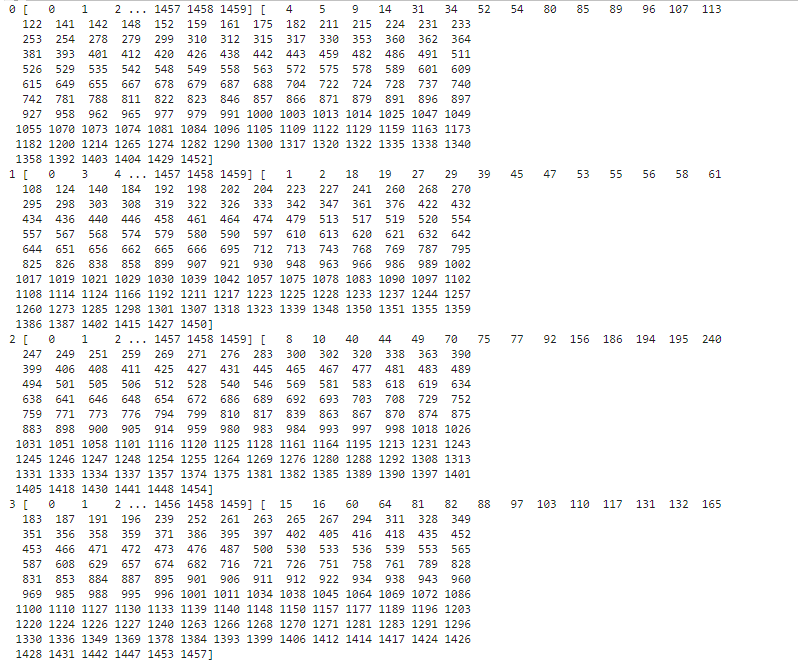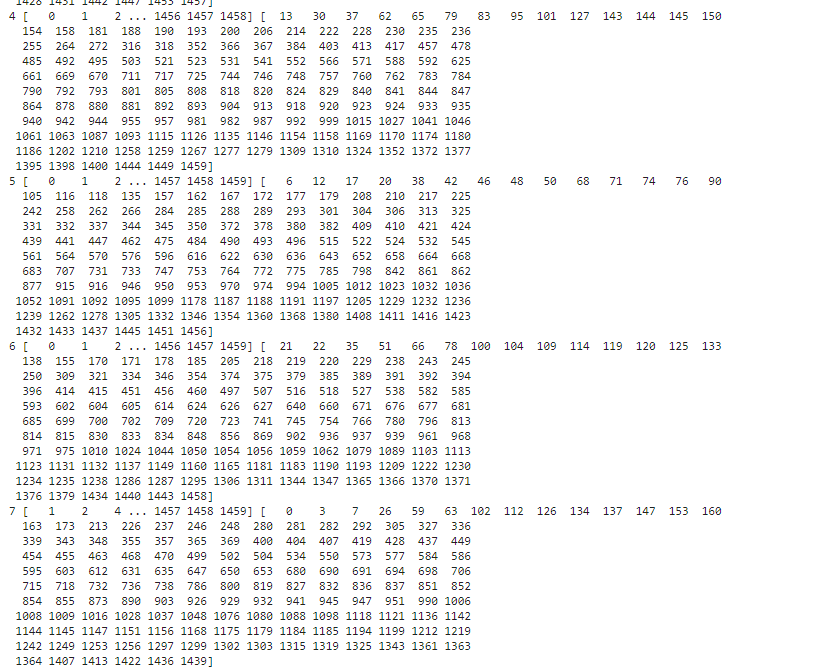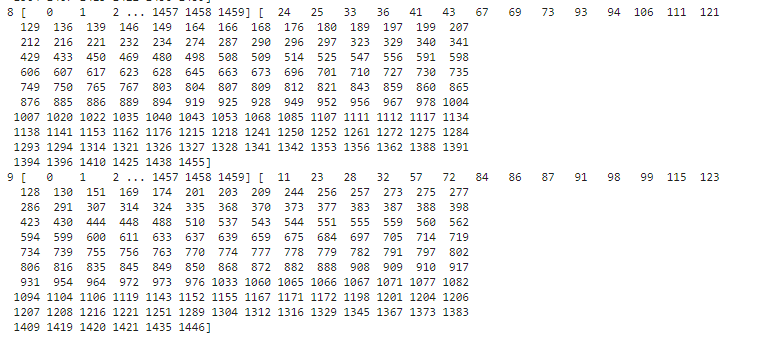``````et_params = {
'n_jobs': 16,
'n_estimators': 100,
'max_features': 0.5,
'max_depth': 12,
'min_samples_leaf': 2,
}

rf_params = {
'n_jobs': 16,
'n_estimators': 100,
'max_features': 0.2,
'max_depth': 12,
'min_samples_leaf': 2,
}

xgb_params = {
'seed': 0,
'colsample_bytree': 0.7,
'silent': 1,
'subsample': 0.7,
'learning_rate': 0.075,
'objective': 'reg:linear',
'max_depth': 4,
'num_parallel_tree': 1,
'min_child_weight': 1,
'eval_metric': 'rmse',
'nrounds': 500
}

rd_params={
'alpha': 10
}

ls_params={
'alpha': 0.005
}
``````

``````xg = XgbWrapper(seed = SEED,params=xgb_params)
et = SklearnWrapper(clf = ExtraTreesRegressor,seed = SEED, params = et_params)#极端随机森林
rf = SklearnWrapper(clf = RandomForestRegressor, seed = SEED, params = rf_params)#随机森林
rd = SklearnWrapper(clf = Ridge, seed = SEED, params = rd_params)#岭回归
ls = SklearnWrapper(clf = Lasso, seed = SEED, params = ls_params)#LASSO回归
``````
``````xg_oof_train, xg_oof_test = get_oof(xg)
et_oof_train, et_oof_test = get_oof(et)
rf_oof_train, rf_oof_test = get_oof(rf)
rd_oof_train, rd_oof_test = get_oof(rd)
ls_oof_train, ls_oof_test = get_oof(ls)
``````
``````print("XG-CV: {}".format(sqrt(mean_squared_error(y_train, xg_oof_train))))
print("ET-CV: {}".format(sqrt(mean_squared_error(y_train, et_oof_train))))
print("RF-CV: {}".format(sqrt(mean_squared_error(y_train, rf_oof_train))))
print("RD-CV: {}".format(sqrt(mean_squared_error(y_train, rd_oof_train))))
print("LS-CV: {}".format(sqrt(mean_squared_error(y_train, ls_oof_train))))
``````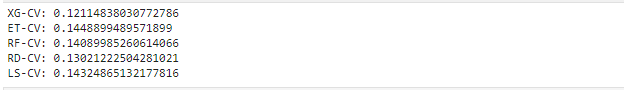``````x_train = np.concatenate((xg_oof_train, et_oof_train, rf_oof_train, rd_oof_train, ls_oof_train), axis=1)
x_test = np.concatenate((xg_oof_test, et_oof_test, rf_oof_test, rd_oof_test, ls_oof_test), axis=1)
``````
``````print("{},{}".format(x_train.shape, x_test.shape))
``````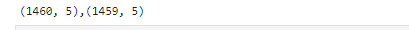``````dtrain = xgb.DMatrix(x_train, label=y_train)
dtest = xgb.DMatrix(x_test)
``````
``````xgb_params = {
'seed': 0,
'colsample_bytree': 0.8,
'silent': 1,
'subsample': 0.6,
'learning_rate': 0.01,
'objective': 'reg:linear',
'max_depth': 1,
'num_parallel_tree': 1,
'min_child_weight': 1,
'eval_metric': 'rmse',
}
``````
``````res = xgb.cv(xgb_params, dtrain, num_boost_round=1000, nfold=4, seed=SEED, stratified=False,
early_stopping_rounds=25, verbose_eval=10, show_stdv=True)
``````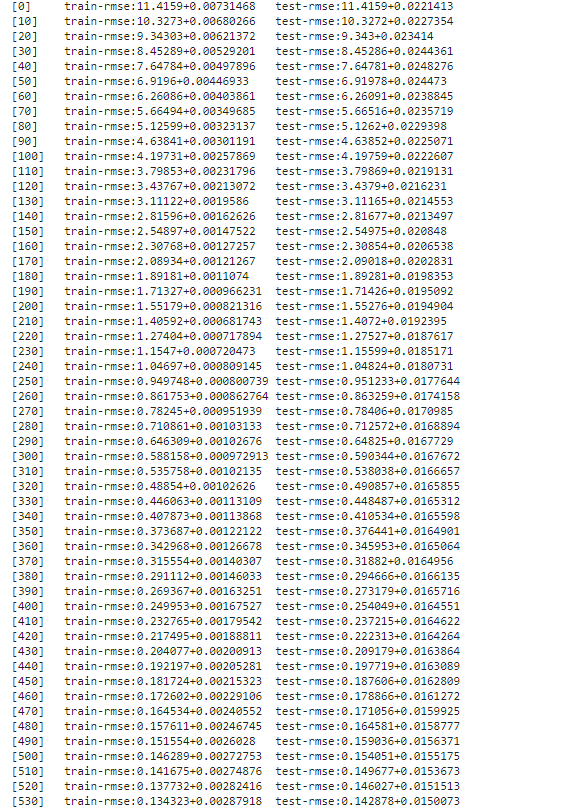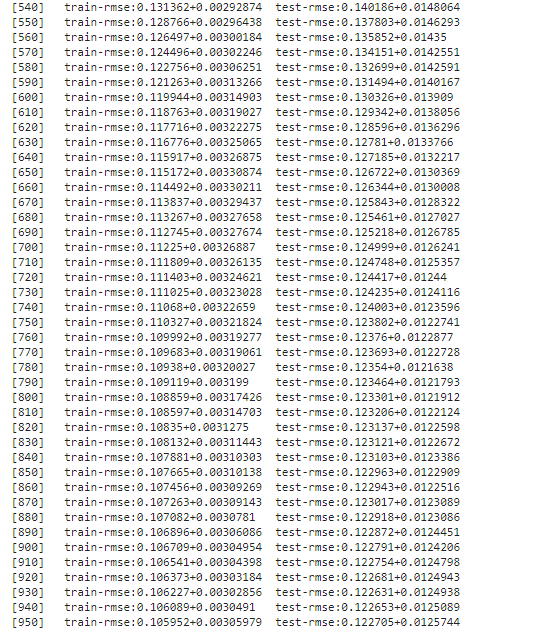``````best_nrounds = res.shape - 1
cv_mean = res.iloc[-1, 0]
cv_std = res.iloc[-1, 1]
``````
``````print('Ensemble-CV: {0}+{1}'.format(cv_mean, cv_std))
``````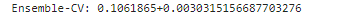``````gbdt = xgb.train(xgb_params, dtrain, best_nrounds)
``````
``````!cat /dev/null > sample_submission.csv#创建相应文件
``````
``````submission = pd.read_csv(SUBMISSION_FILE,engine = 'python',encoding = 'utf-8')
submission.iloc[:,0] = gbdt.predict(dtest)
saleprice = np.exp(submission['SalePrice'])-1
submission['SalePrice'] = saleprice
submission.to_csv('xgstacker_starter.sub.csv', index=None)
``````

##### 三、遇见的问题
###### 1、TypeError: ‘KFold’ object is not iterable：

``````for i, (train_index, test_index) in enumerate(kf):
x_tr = x_train[train_index]
y_tr = y_train[train_index]
x_te = x_train[test_index]
``````

``````for i,(train_index, test_index) in enumerate(kf.split(x_train)):
#train
x_tr = x_train[train_index]#当前循环，当前实验的训练数据
y_tr = y_train[train_index]#当前循环的训练数据标签
#test
x_te = x_train[test_index]#当前循环的测试数据
``````

###### 2. No columns to parse from file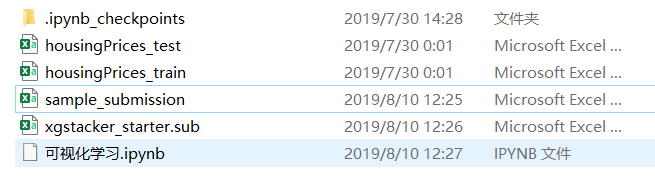python大数据入门 相关内容

2019-01-07 11:03:16 u012080686 阅读数 130
• ###### Python入门视频精讲

Python入门视频培训课程以通俗易懂的方式讲解Python核心技术，Python基础，Python入门。适合初学者的教程，让你少走弯路！ 课程内容包括：1.Python简介和安装 、2.第一个Python程序、PyCharm的使用 、3.Python基础、4.函数、5.高级特性、6.面向对象、7.模块、8.异常处理和IO操作、9.访问数据库MySQL。教学全程采用笔记+代码案例的形式讲解，通俗易懂！！！

8447 人正在学习 去看看 汤小洋

Python在编程语言中数据弱类型语言，变量不需要声明类型，只需要赋值即可，我们就可以了解到该变量为什么类型。在Python中有很多数据类型，天天Python只为大家讲解一些常用常见的数据类型，包括number类型，str字符串类型，list列表类型，tuple元组类型，set集合类型以及dict字典类型。

在讲解数据类型之前，先为大家讲解一个方法type()，type可以用来检测数据类型，当然是用isinstance也可以进行判断检测。为了更直观的得出类型结果，下文会使用type方法。

Number类型：

首先我们想说一些number类型，number其中又包括四个类型：int整型、float浮点数、bool布尔值和complex复数。int整型包含正整数、负整数和0；float浮点数则为小数，0.0也属于浮点数，bool布尔值则只有两个值：Ture和False；complex复数为实数+虚数。我们使用Pythonshell进行number类型的变量赋值和类型检测，如下图所示：

Str字符串：

字符串是Python中最常见的类型之一，变量赋值的时候将值加上引号，则表示字符串，单引号、双引号和三引号均可。其中三种引号可以嵌套使用，为了不产生歧义，尽量不要在嵌套使用时使用同类引号。其中使用var = str(1)进行声明，则var的类型同样为str类型，下图为字符串的几种表达方式及字符串的声明方式：

List列表：

列表类型其实和其他语言中的数组较为类似，Python中也有数组类型，只不过定义的方式不同并且没有list常用。列表顾名思义就是一个队列，将一个或一组数字放到[ ]中，就是list列表类型。大家可以查看下图来了解list列表的声明方式和表达方式：

Tuple元组：

元组与列表类似，也是一些序列的顺序组合，但是组合之后不可以进行修改，元组中每个数据之间也是和列表一样，使用英文逗号隔开。元组还有另外一个特点，就是空元祖，默认的()则为一个元组，还有就是只有一个数据的时候，一个数据后面则需要有一个小逗号，否则会出错。大家可以参考以下代码示例：

Set集合：

set集合类型一组特定数据的无序组合，并且组合内的数据不会重复，这组数据存在{}中，因为集合不会重复，可以用来做去重工作。下面我们通过代码示例对元组进行详细的操作讲解，如下图：

Dict字典：

字典类型和其他的几种数据类型有所区别，同样的使用{}进行表示，但是需要两个变量进行组合成一对才叫做字典，一个叫做键，一个叫做值。虽然都是用{}，但如果是{}中的内容为空，则数据类型依然为字典类型。字典和集合一样，属于无序类型，打印出来的结果随机排序。下图我们进行字典的创建和了解字典的声明方式：

以上数据类型中，list、tuple、set和dict均属于容器类数据，可以放置多个数据内容。Python的数据类型远不止这几种，还有自定义的类型、对象等多种类型。今天所涉及的只是数据类型概念性的内容，等到大家学习更多内容的时候还可以接触到类型转换和相应的函数操作。学习更多Python相关内容，请关注天天Python系列教程--【Python七天入门

python大数据入门 相关内容